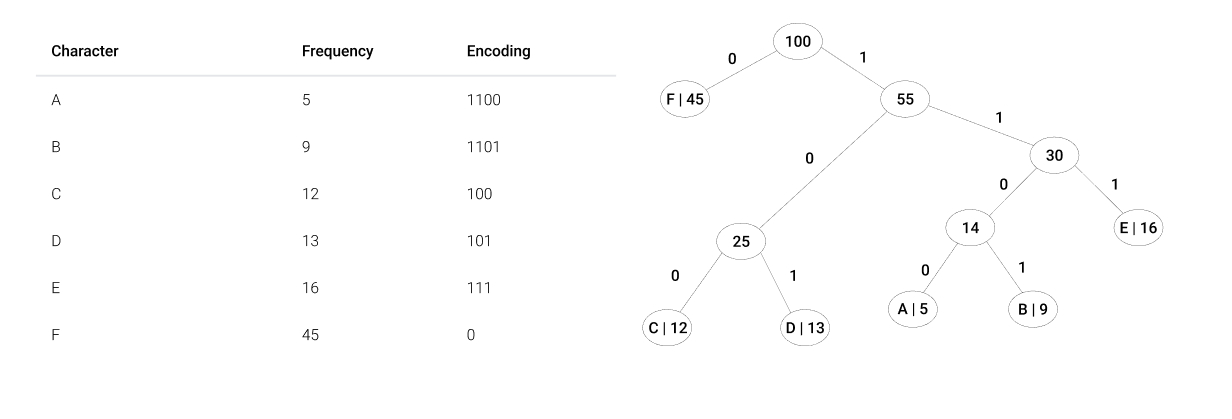# Huffman Coding Algorithm in a Playground

Did you know that you can find a video encoder on GitHub that allows you to turn any single file (exe, doc, etc.) into a video stream? With one hour of video you can upload about 45 GB of data to YouTube. Thus, by setting the videos as private, you can create cloud storage with limited access.

Unbelievable, isn’t it? That’s the power of encoding.

But for now, let’s talk about a famous encoding algorithm developed by David A. Huffman.

Huffman Coding is an algorithm used for compressing data to reduce its size without losing any of its details.

• Greedy-based algorithm: build a prefix tree that optimizes the encoding scheme so that the most frequently used symbols have the shortest encoding.
• Variable-length encoding: it assigns a variable-length code to all the characters in the given stream of data.
• Prefix Rule: the code which is assigned to any character should not be the prefix of any other code to avoid ambiguities.
• Frequency-sorted Binary Tree: this algorithm builds a tree in bottom up manner, where the bottom nodes represent less frequent characters and the root node represent the most used character. The single root node contains data as well as references to left and right nodes (each leaf of the tree corresponds to a letter in the given alphabet). Each left and right nodes can also contain data and references to its own left and right nodes.

# How to build the Huffman tree

1. Create a leaf node for each unique character and its frequency from the given stream of data (build a min heap of all leaf nodes, where min heap is used as a priority queue and the value of frequency field is used to compare two nodes in min heap).

2. Extract the two minimum frequency nodes from the nodes.

3. Create a new internal node with a frequency equal to the sum of the two nodes frequencies. Make the first extracted node as its left child and the other extracted node as its right child. Add this node to the tree.

4. Repeat steps 2. and 3. until the heap contains only one node. The remaining node is the root node and the tree is complete.# And now the Playground

The HuffEncoder app is for iOS and the goal is to compress and decompress a selected .txt file, using the Huffman Coding Algorithm.

The HuffEncoder is an app playground for compressing text inputs using the Huffman Coding Algorithm, and displays the size of the compressed and decompressed data using Swift Charts.# Huffman Coding Time Complexity

• Priority Queue: the time taken for encoding each given unique character depending on its frequency is O(n log n), where n is the number of nodes.

• Tree Array: iterating to find the next two smallest nodes, the time taken for the compressor becomes O(n^2).

# Some applications of Huffman Coding

• Image compression.

• Multimedia storage like JPEG, PNG, and MP3.

• Data transmission using fax and text.

# Resources used:

https://www.scaler.com/topics/huffman-coding/

https://victorqi.gitbooks.io/swift-algorithm/content/huffman_coding.html

https://www.geeksforgeeks.org/huffman-coding-greedy-algo-3/

https://brilliant.org/wiki/binary-search-trees/

https://brilliant.org/wiki/huffman-encoding/

https://www.dsi.unive.it/~labasd/lezioni/lezione09/lezione9.pdf

https://github.com/DvorakDwarf/Infinite-Storage-Glitch

View Github